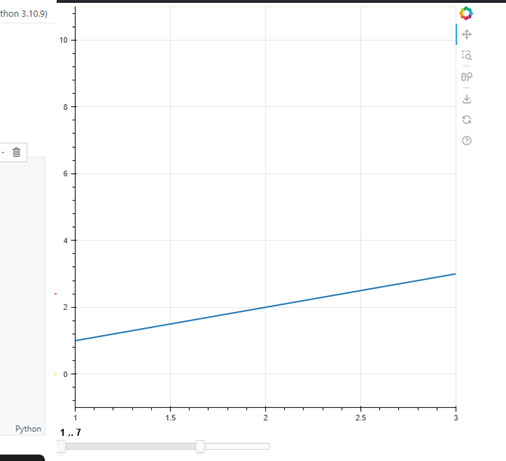# Creating a simple Range Slider in Bokeh

Bokeh is a powerful data visualization library in Python that helps to create interactive and unique visualizations for the web. Bokeh supports various rendering techniques and provides a wide range of built-in tools for creating complex visualizations with multiple glyphs. This document will guide you through the process of creating a plot with multiple glyphs using Bokeh. This plot combines different glyphs to display multiple data series in a single plot that provides a more efficient way to understand the relationship between different variables.

## What are the key benefits of range slider?

• Interactive − RangeSlider provides an interactive way for users to adjust the range of a plot, which can be particularly useful for exploring data and identifying trends.

• Range control − The RangeSlider allows users to control the range of the data displayed on a plot, making it easier to zoom in on specific areas of interest.

• Easy to use − RangeSlider is easy to use and can be added to a Bokeh plot with just a few lines of code.

• Customizable − RangeSlider can be customized in terms of appearance and behavior, allowing developers to create sliders that fit their specific needs.

• Integration with other widgets − RangeSlider can be integrated with other Bokeh widgets to create more complex and interactive visualizations.

## Prerequisites

Before we dive into the task few things should is expected to be installed onto your system −

List of recommended settings −

• pip install pandas, bokeh

• It is expected that the user will have access to any standalone IDE such as VS-Code, PyCharm, Atom or Sublime text.

• Even online Python compilers can also be used such as Kaggle.com, Google Cloud platform or any other will do.

• Updated version of Python. At the time of writing the article I have used 3.10.9 version.

• Knowledge of the use of Jupyter notebook.

• Knowledge and application of virtual environment would be beneficial but not required.

• It is also expected that the person will have a good understanding of statistics and mathematics.

## Step-by-Step Guide

### Importing Bokeh and relevant libraries

We start by importing the necessary libraries. We will be using bokeh.plotting to create a figure and bokeh.layouts to arrange the slider and the figure.

### Syntax

from bokeh.plotting import figure
from bokeh.layouts import layout
import numpy as np
from bokeh.models import Range1d
from bokeh.models.widgets import RangeSlider
from bokeh.io import show


### Creating Data

Create data for the slider by defining start and end values for the range. We will use a NumPy array to specify the range values.

### Syntax

start = 1
end = 10
values = np.arange(start, end+1, 1)


### Creating a RangeSlider

Now, we will create a RangeSlider object by passing in the range values, initial range and step size. We will set the minimum and maximum range values for the slider.

### Syntax

range_slider = RangeSlider(start=start, end=end, step=1, value=(start, end))


### Creating a Figure

Next, we create a figure object and add a line graph. We will then specify the range and domain of the line graph and position it on the page using a layout object.

### Syntax

plot = figure()
plot.line(x=[1,2,3], y=[1,2,3], line_width=2)
plot.x_range = Range1d(1, 3)
plot.y_range = Range1d(-1, end+1)


### Creating a Layout

Finally, we arrange the slider and the figure using a layout object.

### Syntax

layout = layout([[plot], [range_slider]])


### Displaying the Slider and Figure

To view the slider and the figure, we use the show function.

### Syntax

show(layout)yout = layout([[plot], [range_slider]])


### Example

from bokeh.plotting import figure
from bokeh.layouts import layout
import numpy as np

start = 1
end = 10
values = np.arange(start, end+1, 1)

from bokeh.models.widgets import RangeSlider

range_slider = RangeSlider(start=start, end=end, step=1, value=(start, end))
plot = figure()
plot.line(x=[1,2,3], y=[1,2,3], line_width=2)
plot.x_range = range_slider
plot.y_range = (-1, end+1)

layout = layout([[plot], [range_slider]])
from bokeh.io import show
show(layout)


The output for the above code snippet will produce a range slider with the values 1 to 10.

## Final Program,Code

from bokeh.plotting import figure
from bokeh.layouts import layout
import numpy as np
from bokeh.models import Range1d
from bokeh.models.widgets import RangeSlider
from bokeh.io import show

start = 1
end = 10
values = np.arange(start, end+1, 1)

range_slider = RangeSlider(start=start, end=end, step=1, value=(start, end))
plot = figure()
plot.line(x=[1,2,3], y=[1,2,3], line_width=2)
plot.x_range = Range1d(1, 3)
plot.y_range = Range1d(-1, end+1)

layout = layout([[plot], [range_slider]])
show(layout)


### OutputIn the above section we can see the output of the range slider in bokeh and also example for better understanding.

## Conclusion

In this tutorial, you have learned how to create a simple range slider in Bokeh. You created a RangeSlider, a Figure, and a Layout, to display a range of values in a line chart while allowing the user to select a range of values. Bokeh provides a lot of flexibility and customization options to create dynamic and interactive data visualizations. This tutorial serves as a starting point to build more complex interactive visualizations with Bokeh.

Updated on: 25-Apr-2023

348 Views